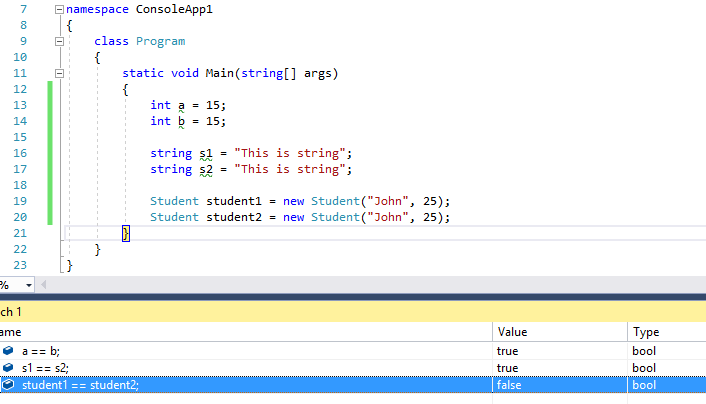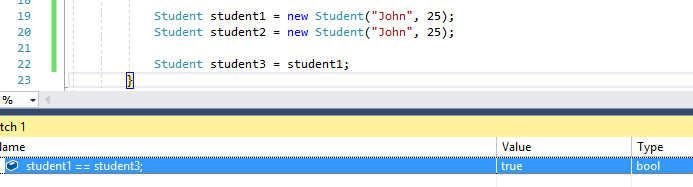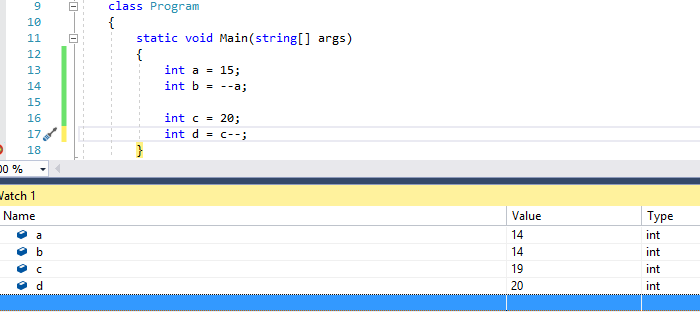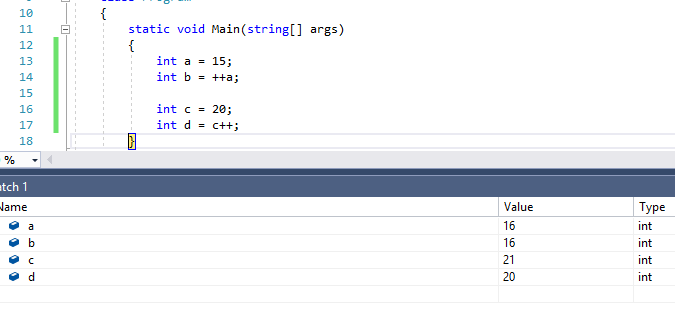In this article, we are going to talk about operators in C#, how to use them and what are the differences between each of them.

For the complete navigation of this series check out: C# Back to Basics.

## Type of Operators

The most used operators in C# are:## Arithmetic Operators

Arithmetic operators are defined for all the numeric data types. Operators +, -, *, / represent the basic binary arithmetic operations (addition, subtraction, multiplication and division). Operator % is the remainder after division. The important thing to notice is that the + operator behaves differently with the number and string types. With the numbers, the result of expression 5 + 5 is 10. But with the strings, the result of expression „5“ + „5“ is „55“. So with the number type, it is an addition operator but with the string type, it is a concatenation operator.

## Relational Operators

All the relational operators return a true or false result. They are used to compare expressions or variables from both sides of the relational operator. These operators have a lower priority than arithmetic ones. So in the following example: `x*a-8*b>y+5*z;` the left side of the greater than operator has calculated first then the right side and then they are compared.

For the value-type variables and the strings, the `==` (equality) operator will return true only if they are the same, otherwise, it will return false. But if variables are reference types then the `==` operator will return true only if those two variables point to the same memory location, otherwise, it will return false.

So let’s see this through an example:We see that `a` and` b` are the equal as well as the `s1` and `s2`. But the `student1` and `student2` are not equal because they point to different memory locations. But if we create another variable of type `Student` and assign the value of `student1` variable to it, the `==` operator will return true:## Logical Operators

The logical operators `&&` (and) and `||` (or) serve to connect logical values. Expression `<expression1>&&<expression2>` is true only if both expressions are true. Expression `<expression1>||<expression2>` is false only if both expressions are false, otherwise, it is true.

The `!` (negation) operator negates the logical value it is applied on. It has the highest priority of all the operators mentioned. So the expression `!logicalValue` will be false only if logicalValue is true and vice verse.

Let’s see this with an example:

```class Program
{
static void Main(string[] args)
{
int a = 14;
int b = 30;
int c = 20;

if(a < b && a < c)
{
Console.WriteLine(\$"min number is {a}");
}

if(a < b || a < c)
{
Console.WriteLine("The a variable is smaller then b or c");
}

if(!(a > b))
{
Console.WriteLine("a is less than b");
}
}
}
```

## Increment and Decrement Operators

In the C# language, we can use operators that increments and decrements the variable value by 1. Those operators are `++` and `--`, and they are very useful in many cases. So, the better way of writing this code:

```static void Main(string[] args)
{
int a = 15;
a = a + 1; //now it is 16
}
```

Would be to write it like this:
```static void Main(string[] args)
{
int a = 15;
a++; //now it is 16
}
```

The same applies to the `--` operator.

These two operators have the prefix notations: –variable, ++variable and the suffix notations: variable–, variable++ . Even though both notations will change the value by 1, the result will be different. This is easier to explain through an example:What we can notice is that the prefix notation decrements the value of `"a"` variable first and then assigns that value to the `"b"` variable. But the expression with suffix notation is different. The value of the `"c"` variable is assigned to the `"d"` variable first and then decremented by 1.

The same applies for the increment operator:## Conclusion

Excellent. Now we have more knowledge about operators in C#. In the next part, we are going to talk about the type-conversions in C#.

In the next post, we are going to talk about type conversions in C#.